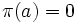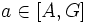# Centralizer-commutator product decompoition for abelian groups

## Statement

Suppose$A$ is an Abelian group and$G \le \operatorname{Aut}(A)$ is a finite group such that the map$a \mapsto |G|a$ is a bijective map on$A$ (when$A$ is finite, this is equivalent to requiring that the orders of$A$ and$G$ be relatively prime). Define:

•$[A,G]$ is the subgroup generated by elements of$A$ of the form$g(a) - a$, where$a \in A, g \in G$.
•$C_A(G)$ is the subgroup comprising those$a \in A$ such that$g(a) = a$.

Note that these correspond to the usual notions of commutator of two subgroups and centralizer if we look inside the semidirect product of$A$ by$G$.

Then,$A$ is the internal direct product of the subgroups$[A,G]$ and$C_A(G)$. In other words, we have:$A = [A,G] \times C_A(G)$.

## Proof

Given: A finite Abelian group$A$, a group$G \le \operatorname{Aut}(A)$ such that the orders of$A$ and$G$ are relatively prime.

To prove:$A = [A,G] \times C_A(G)$

Proof: We define a map$\pi: A \to A$, prove that$\pi$ is a projection, and show that the kernel and image of$\pi$ are$[A,G]$ and$C_A(G)$ respectively.

Define:$\pi(a) = \frac{1}{|G|} \sum_{g \in G} g(a)$.

Note that this expression makes sense because the multiplication by$|G|$ map is invertible in$A$ by the condition of relatively prime orders.

We observe that:$\pi \circ g = g \circ \pi = \pi \ \forall \ g \in G$.

This is because$\pi \circ g$ and$g \circ \pi$ yield the same summation as$\pi$, in a different order, and the group is Abelian.

This further yields:$\pi^2 = \pi$.

Thus,$\pi$ is an idempotent endomorphism of$A$. In other words, the image of$\pi$ equals its fixed-point space.

1. Computation of the image of$\pi$:
1.$C_A(G)$ is in the image: If$a \in C_A(G)$, then$g(a) = a$ for all$g \in G$, so$\pi(a) = a$. Thus,$\pi(a) = a$, so$C_A(G)$ is in the fixed-point space of$\pi$.
2. The image is in$C_A(G)$: If$a$ is fixed under$\pi$, then$a = \pi(a) = g(\pi(a)) = g(a)$, so$a$ is fixed by all$g \in G$. Thus, the fixed-point space of$\pi$, which is also the image of$\pi$, is precisely$C_A(G)$.
2. Computation of the kernel of$\pi$:
1.$[A,G]$ is in the kernel: Let's now consider the kernel of$\pi$. Suppose$a = g(b) - b$ for some$b \in A$. Then$\pi(a) = \pi(g(b)) - \pi(b) = \pi(b) - \pi(b) = 0$. Thus, any element in$[A,G]$ is in the kernel of$\pi$.
2. The kernel is in$[A,G]$: If$\pi(a) = 0$, then$a = - \frac{1}{|G|} \sum_{g \in G} g(a) - a$. This yields that$a \in [A,G]$. Thus, the kernel of$\pi$ is precisely$[A,G]$.